The corresponding files can be obtained from:

Linear thermoelasticity (weak coupling)¶

Introduction¶

In this tour, we will solve a linear thermoelastic problem. In permanent regime, the temperature field is uncoupled from the mechanical fields whereas the latter depend on the temperature due to presence of thermal strains in the thermoelastic constitutive relation. This situation can be described as weak thermomechanical coupling. Full thermomechanical coupling for a transient evolution problem is treated in this tour.

In the present setting, the temperature field can be either given as a Constant or an Expression throughout the domain or obtained as the solution of a steady-state heat (Poisson) equation. This last case will be implemented in this tour.

Problem position¶

We consider the case of a rectangular 2D domain of dimensions $$L\times H$$ fully clamped on both lateral sides and subjected to a self-weight loading. The bottom side is subjected to a uniform temperature increase of $$\Delta T = +50^{\circ}C$$ while the top and lateral boundaries remain at the initial temperature $$T_0$$. The geometry and boundary regions are first defined.

:
from dolfin import *
from mshr import *
import matplotlib.pyplot as plt
%matplotlib notebook

L, H = 5, 0.3
mesh = RectangleMesh(Point(0., 0.), Point(L, H), 100, 10, "crossed")

def lateral_sides(x, on_boundary):
return (near(x, 0) or near(x, L)) and on_boundary
def bottom(x, on_boundary):
return near(x, 0) and on_boundary
def top(x, on_boundary):
return near(x, H) and on_boundary

Because of the weak coupling discussed before, the thermal and mechanical problem can be solved separately. As a result, we don’t need to resort to Mixed FunctionSpaces but can just define separately both problems.

Resolution of the thermal problem¶

The temperature is solution to the following equation $$\text{div}(k\nabla T) = 0$$ where $$k$$ is the thermal conductivity (here we have no heat source). Since $$k$$ is assumed to be homogeneous, it will not influence the solution. We therefore obtain a standard Poisson equation without forcing terms. Its formulation and resolution in FEniCS is quite standard with the temperature variation $$\Delta T$$ as the main unknown.

Note: We needed to define the linear form LT in order to use the standard solve(aT == LT, T, bcT) syntax for linear problems. We could also have equivalently defined the residual res = dot(grad(T), grad(T_))*dx and used the nonlinear-like syntax solve(res == 0, T, bcT).
:
VT = FunctionSpace(mesh, "CG", 1)
T_, dT = TestFunction(VT), TrialFunction(VT)
Delta_T = Function(VT, name="Temperature increase")
LT = Constant(0)*T_*dx

bcT = [DirichletBC(VT, Constant(50.), bottom),
DirichletBC(VT, Constant(0.), top),
DirichletBC(VT, Constant(0.), lateral_sides)]
solve(aT == LT, Delta_T, bcT)
plt.figure()
p = plot(Delta_T, mode="contour")
plt.colorbar(p)
plt.show()
Calling FFC just-in-time (JIT) compiler, this may take some time.
Calling FFC just-in-time (JIT) compiler, this may take some time.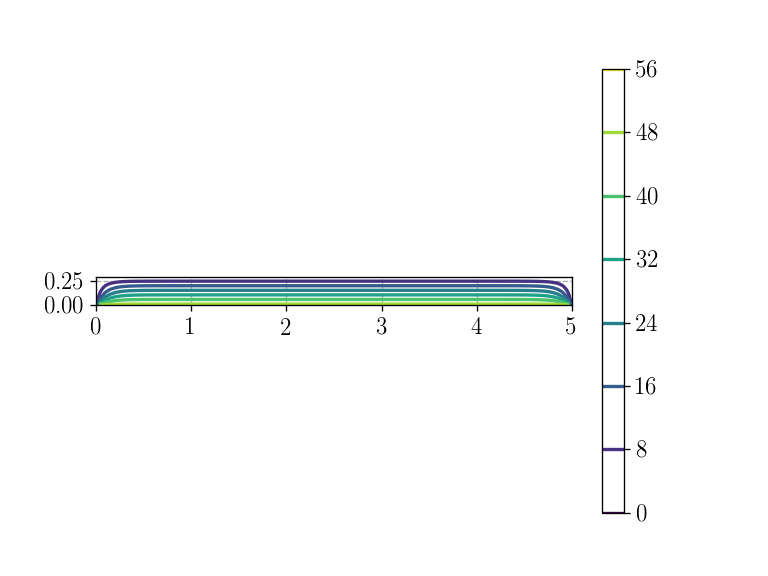Mecanical problem¶

The linearized thermoelastic constitutive equation is given by:

$\begin{equation} \boldsymbol{\sigma} = \mathbb{C}:(\boldsymbol{\varepsilon}-\alpha(T-T_0)\boldsymbol{1}) = \lambda\text{tr}(\boldsymbol{\varepsilon})\boldsymbol{1}+2\mu\boldsymbol{\varepsilon} -\alpha(3\lambda+2\mu)(T-T_0)\boldsymbol{1} \end{equation}$

where $$\lambda,\mu$$ are the Lamé parameters and $$\alpha$$ is the thermal expansion coefficient. As regards the current problem, the last term corresponding to the thermal strains is completely known. The following formulation can thus be generalized to any kind of known initial stress or eigenstrain state such as pre-stress or phase changes.

The weak formulation for the mechanical problem builds upon the presentation of the 2D linear elasticity tour. The main difference lies in the presence of the temperature term in the constitutive relation which introduces a linear term with respect to the TestFunction u_ when writing the work of internal forces inner(sigma(du, Delta_T), eps(u_))*dx. As a result, the bilinear form is extracted using the left-hand side lhs function whereas the thermal strain term, acting as a loading term, is extracted using the right-hand side rhs function and added to the work of external forces when defining the linear form LM.

:
E = Constant(50e3)
nu = Constant(0.2)
mu = E/2/(1+nu)
lmbda = E*nu/(1+nu)/(1-2*nu)
alpha = Constant(1e-5)

f = Constant((0, 0))

def eps(v):
def sigma(v, dT):
return (lmbda*tr(eps(v))- alpha*(3*lmbda+2*mu)*dT)*Identity(2) + 2.0*mu*eps(v)

Vu = VectorFunctionSpace(mesh, 'CG', 2)
du = TrialFunction(Vu)
u_ = TestFunction(Vu)
Wint = inner(sigma(du, Delta_T), eps(u_))*dx
aM = lhs(Wint)
LM = rhs(Wint) + inner(f, u_)*dx

bcu = DirichletBC(Vu, Constant((0., 0.)), lateral_sides)

u = Function(Vu, name="Displacement")

:
solve(aM == LM, u, bcu)

plt.figure()
p = plot(1e3*u,title="Vertical displacement [mm]")
plt.colorbar(p)
plt.show()
plt.figure()
p = plot(sigma(u, Delta_T)[0, 0],title="Horizontal stress [MPa]")
plt.colorbar(p)
plt.show()
Calling FFC just-in-time (JIT) compiler, this may take some time.
Calling FFC just-in-time (JIT) compiler, this may take some time.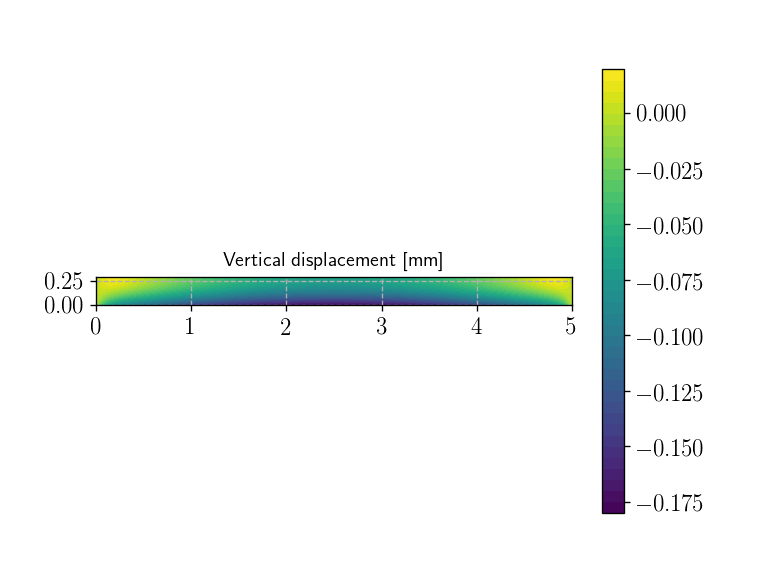Calling FFC just-in-time (JIT) compiler, this may take some time.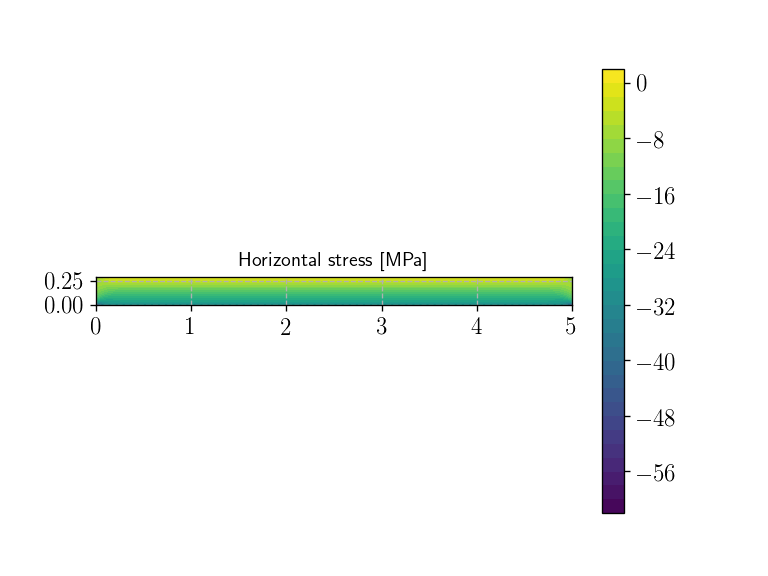Calling FFC just-in-time (JIT) compiler, this may take some time.

We now take into account the self-weight.

:
rho_g = 2400*9.81e-6
f.assign(Constant((0., -rho_g)))
solve(aM == LM, u, bcu)

plt.figure()
p = plot(1e3*u,title="Vertical displacement [mm]")
plt.colorbar(p)
plt.show()
plt.figure()
p = plot(sigma(u, Delta_T)[0, 0],title="Horizontal stress [MPa]")
plt.colorbar(p)
plt.show()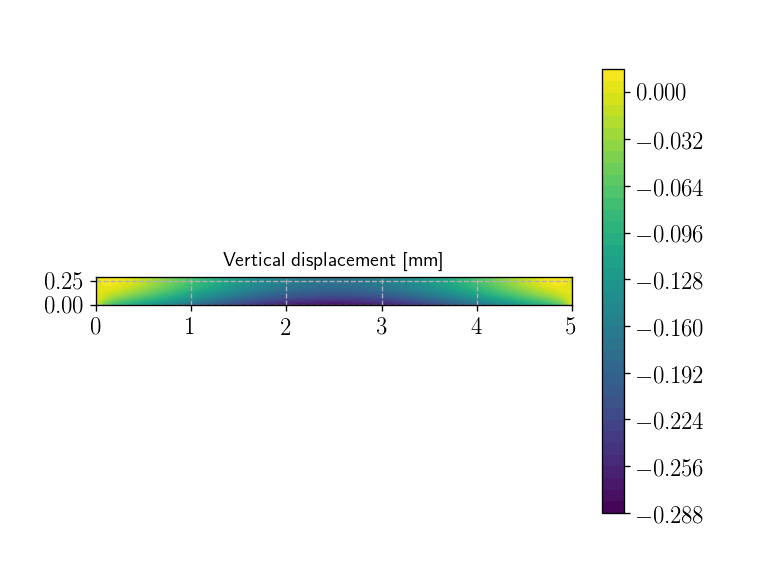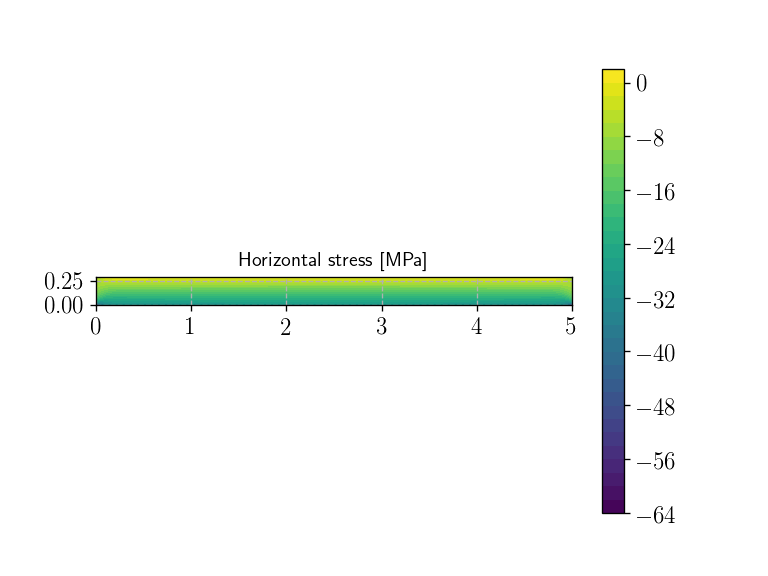[ ]: